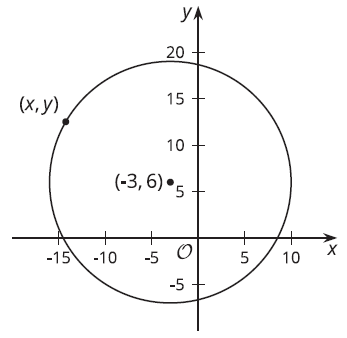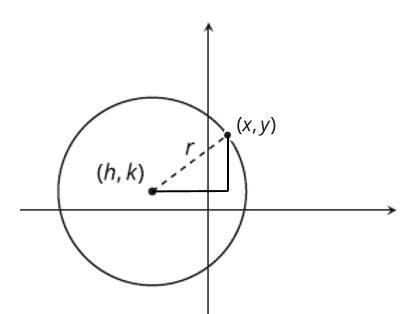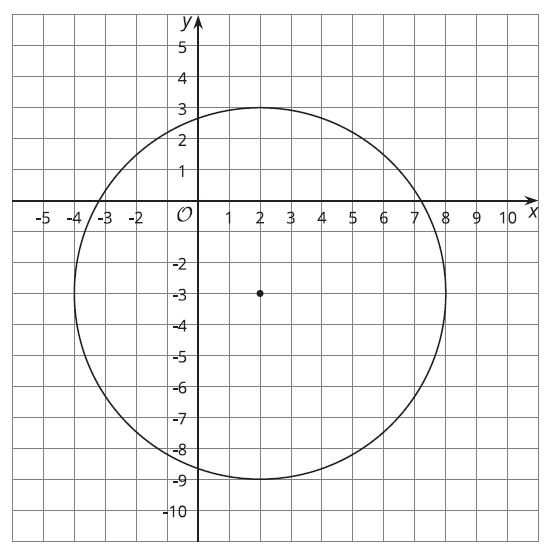# G.6.4.3 Building an Equation for a Circle

Topic:
Circle

We traditionally use the letter r to stand for the radius of a circle, and we use the letters (h, k) to stand for the center of a circle. The image shows a circle with center (-3, 6) and radius 13 units.Write an equation that would allow you to test whether a particular point (x, y) is on the circle.

Use your equation to test whether (9, 1) is on the circle.

Let's generalize the equation you'd use to test whether a particular point (x, y) is on the circle.Use (x, y) and (h, k) to write the length of each leg of the triangle. Then use the pythagorean theorem to write an equation. This will be the equation that defines a circle (just like y = mx + b is the equation that defines a line).

Write an equation for circle below that has center at (2, -3) and a radius of 6.